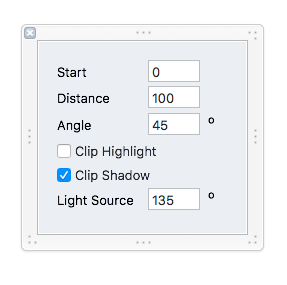# Adding points at specified angle

Is it possible to get a more low-level function in addition to `- (void)addExtremePoints;`? So instead of checking against 0 and 90 degrees, one could search for an arbitrary angle and add points there.

``````- (void)addPointsAtAngle:(CGFloat)AngleInRadians;
``````

## Maybe Irrelevant Motivation

I’m hoping to automate a 3d effect à la https://glyphsapp.com/tutorials/creating-a-layered-color-font with a filter.

Curved path segments like the bottom right corner of the `U` above are the pain point here, since you need to have a node on that path segment where the tangent is (e.g.) 45 degrees. and then extrude that node at 45 degrees.

Ultimately this filter might have a dialog like:Feedback welcome on filter features.

You can rotate by an angle and add extremes, and then rotate back.

1 Like

I couldn’t find a solution for this as well, so I wrote a little script which will do just that. It doesn’t have an interface yet, but I plan to add one before I publish this on GitHub.

Please bear in mind I don’t know calculus so I came up with a solution to brute force it. This is far from perfect and a highly error-prone method but it works for meAny feedback to improve it is much appreciated.

``````from collections import OrderedDict

# Set the angle
angle = 135
# Calculates the opposite angle as well
secondAngle = angle + 180 if angle <= 90 else angle - 180
angles = [ angle, secondAngle ]

# Set the time step which will be used to check the tangents.
# On a path, time represents the position of a "sweep" between
# two oncurve nodes. Smaller values mean more precision,
# but also take more time to run. I get good results with 0.005.
timeStep = 0.005

font = Glyphs.font
layer = font.selectedLayers

for path in layer.paths:

# Creates a list of indexes of oncurve nodes.
# Only oncurve nodes can be used to sweep a path using time.
onCurveNodes = []
for node in path.nodes:
if node.type != "offcurve":
onCurveNodes.append( node.index )

# Sweeps the whole path to find where the tangent angle matches
# the angles we're searching for. The points where the tangent angle
# match are stored in a dict. This is probably very, VERY inefficient
# but I don't know calculus :(
tangentPoints = {}
for nodeTime in onCurveNodes:
endTime = nodeTime + 1
while nodeTime < endTime:
# Creates a temporary path so we don't mess with the original
# until we find the correct point.
tempPath = layer.paths.copy()
# Creates a node in our fake path at the current time...
nearestNode = tempPath.insertNodeWithPathTime_( nodeTime )
# ... so we can get its tangent angle.
tangentAngle = tempPath.tangentAngleAtNode_direction_(nearestNode, 1)
# If the tangent angle matches, store the current time in the dict
# and skip the rest of this segment.
if int(tangentAngle) in angles:
tangentPoints[nodeTime] = nearestNode
break
nodeTime += timeStep

# Now, creates an ordered dict from the results.
# This dict is sorted from largest to smallest time so we can simply insert
# the nodes, not worrying about their new indexes.
orderedPoints = OrderedDict( sorted(tangentPoints.items(), reverse=True) )
for time, point in orderedPoints.iteritems():
# Insert the new nodes and profit!
path.insertNodeWithPathTime_( time )
``````

The easiest way to do this is to rotate the segment by the angle you are looking for and then find the extreme points (you have to ignore the horizontal or vertical extremes, depending how you rotated). Then rotate them back.